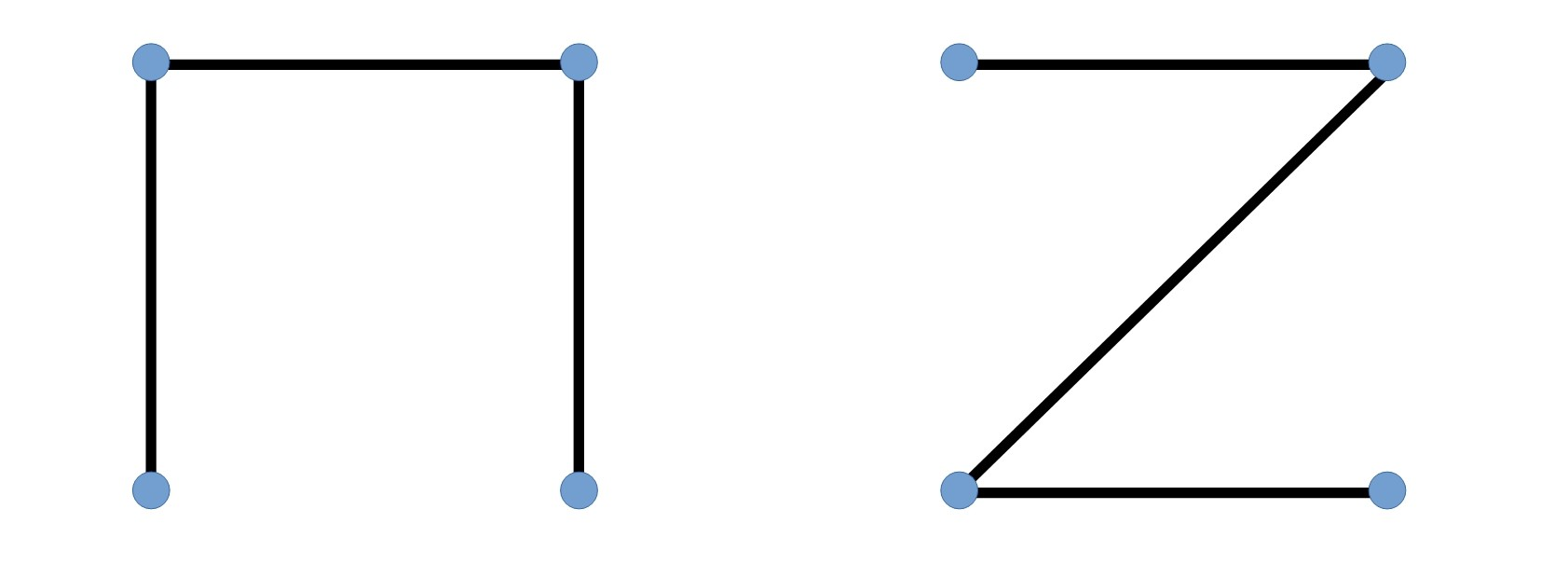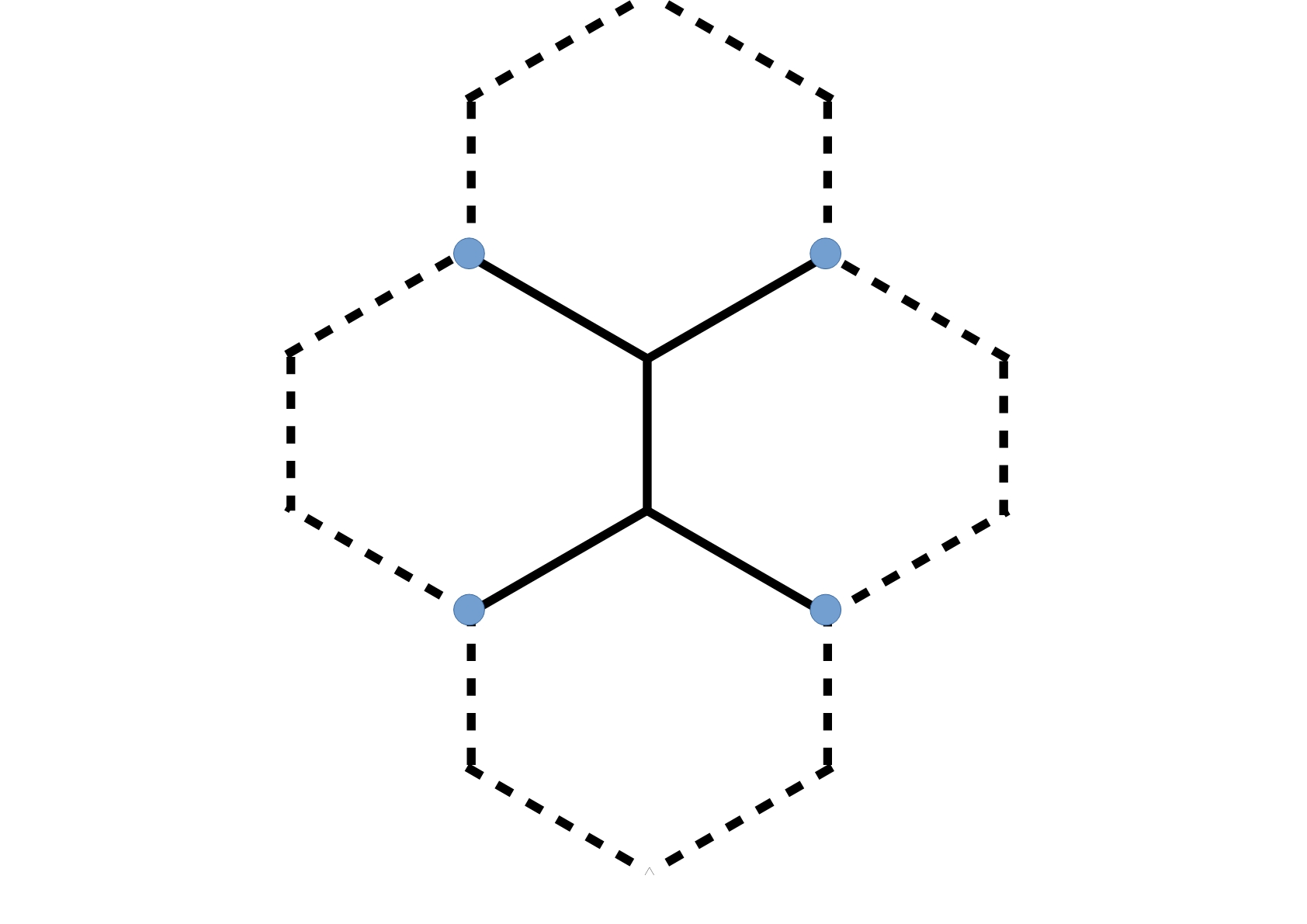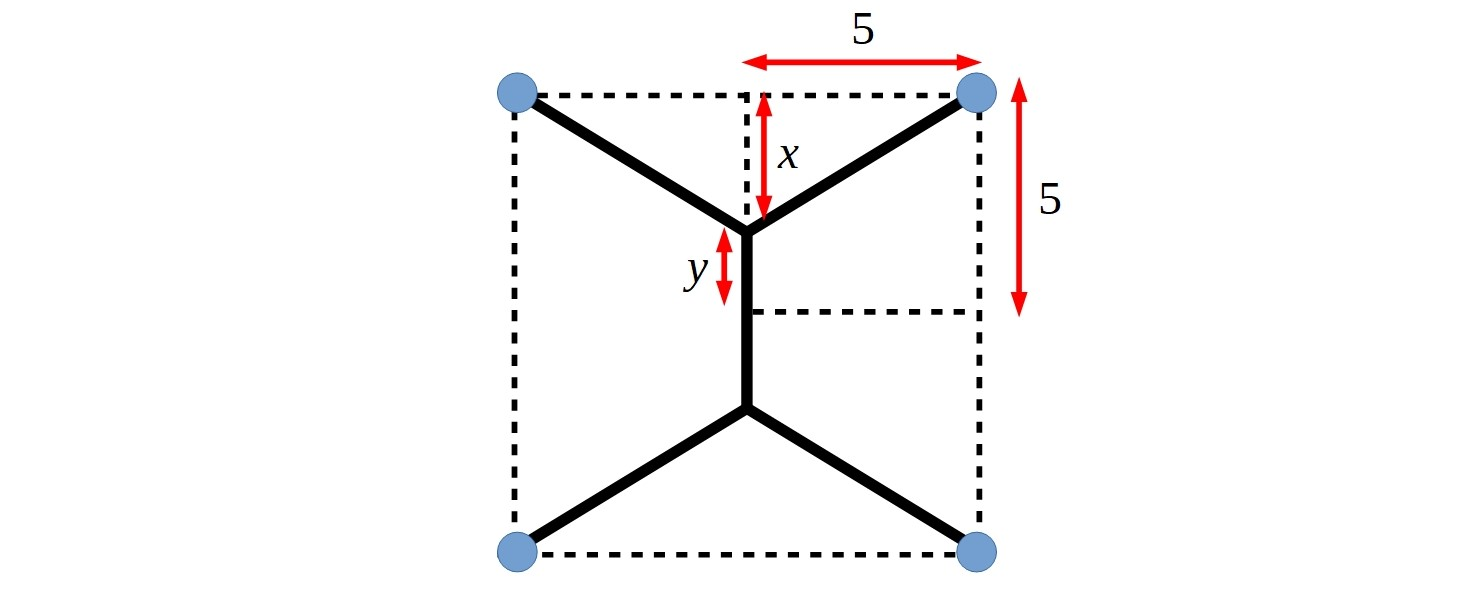#### You may also like### Frosty the Snowman

Frosty the Snowman is melting. Can you use your knowledge of differential equations to find out how his volume changes as he shrinks?### A Frosty Puddle

Can you draw a sketch of how Frosty's volume changes as his height decreases?### Frosty Is Melting!

Frosty the Snowman is melting. Can you work out how much less snow he contains when he is at half his starting height?

# Minimal Connector

##### Age 14 to 18Challenge Level

There are a variety of contexts in which it is important to be able to minimise the length of a network joining some fixed points, in order to minimise the use of resources or energy.  This might be trying to create a gas pipeline connecting towns or creating a fibre-optic cable network.

Points on the network do not need to be connected directly to each other, but you must be able to get from any point to any other point on the network. The smaller the network is, the greater the environmental saving and the greater the efficiency of the system.

Imagine four points (they could be houses, or towns, or data points) arranged at the corners of a square of side length 10km.

Here are two possible ways to connect the four points:What is the total length of the network in each case?  Can you find a better solution?

What is the minimum network that can connect them?
(A solution of less than 28km is possible!)

Charlie took inspiration from honeycombs to wonder if hexagons might be helpful.  Click the button below to see what Charlie was thinking about.  Can you use this to find a shorter path?Claire remembered seeing bubbles made inside cubes, and thought that the minimum network might look similar.  Click the button below to see what she thought the best solution might look like.You can find out how to make bubbles inside cubes with this video from The Science Museum Group.

Both Alf and Claire used this idea to try and answer the question.

Alf thought that he might be able to use a spreadsheet to help him find a better solution.  Click the button below to see his first spreadsheet.  Can you use this idea to find the best solution?Claire has learnt some Calculus techniques and thought she could use this to prove that she had the minimum length.  If you have learnt some Calculus you might like to try to recreate her solution.

Claire started by finding an expression for the total length, $L$, of the network in terms of $x$.
Then she differentiated this to find $\frac{\mathrm{d} L}{\mathrm {d} x}$, and used this to find the value of $x$ where this is equal to 0.
Then she used this value of $x$ to find the minimum value of $L$.
Finally she used Desmos to sketch a graph of $L$ to check her answer.

Do you get the same answer using all three approaches? Which approach do you prefer?

Once you have tried this problem, you might like to watch this video from James Grime where he uses bubble mixture to find the solution to this problem.  He also shows how this can be adapted to other problems!

This problem is based on a problem from the book Teaching Mathematics as if the Planet Matters